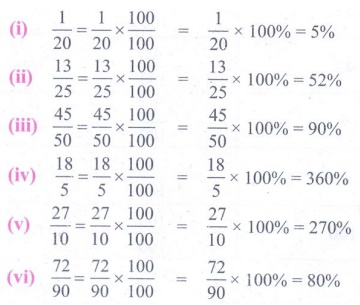Home | | Maths 7th Std | Converting Fraction to Percentage

# Converting Fraction to Percentage

All numbers which are represented using numerator and denominator are fractions.

Converting Fraction to Percentage

All numbers which are represented using numerator and denominator are fractions. They can have any number as a denominator. If the denominator of the fraction is hundred then it can be very easily expressed as a percentage. Let us try to convert different fraction to percentage.

Example 2.1

Write 1/5 as per cent.

SolutionWe have 1/5 = 1/5 × 100/100 = 1/5 ×100% = 100/5 % = 20% .

Example 2.2

Convert 7/4 to per cent.

SolutionWe have 7/4 = 7/4 × 100/100 = 7/4 ×100% = 700/4 % = 175% .

Example 2.3

Out of 20 beads, 5 beads are red. What is the percentage of red.

SolutionWe have =5/20×5/20×100/100 =5/20 ×100% =500/20 % = 25%.

Example 2.4

Convert the fraction 23/30 as per cent.

SolutionWe have 23/30 = 23/30 × 100/100 = 23/30 ×100% = 76 2/3 %

From these examples we see that the percentage of proper fractions are less than 100 and that of improper fractions are more than 100.

Try these

Convert the fractions as percentage.

(i) 1/20  (ii) 13/25   (iii) 45/50   iv 18/5   (v) 27/10  (vi) 72/90

Solution:(i) 1/20 = 1/20 × 100/100 = 1/20 × 100% = 5 %

(i) 1/20 = 1/20 × 100/100 = 1 / 20 × 100% = 5 %

(ii) 13/25 = 13/25 × 100/100 = 13 / 25 × 100% = 52 %

(iii) 45/20 = 45/50 × 100/100 = 45 / 50 × 100% = 90 %

(iv) 18/5 = 18/5 × 100/100 = 18 / 5 × 100% = 360 %

(v) 27/10 = 27/10 × 100/100 = 27 / 10 × 100% = 270 %

(vi) 72/90 = 72/90 × 100/100 = 72 / 90 × 100% = 80 %

Tags : Term 3 Chapter 2 | 7th Maths , 7th Maths : Term 3 Unit 2 : Percentage and Simple Interest
Study Material, Lecturing Notes, Assignment, Reference, Wiki description explanation, brief detail
7th Maths : Term 3 Unit 2 : Percentage and Simple Interest : Converting Fraction to Percentage | Term 3 Chapter 2 | 7th Maths